DPL 9 Help

Beta DistributionBeta Distribution

The Beta Distribution is one of the few named distributions which can be used for a variable confined to a fixed range. As a result, the Beta Distribution is used in a wide variety of applications. It is often used to represent the distribution of the fraction or proportion of events that might occur. One such example would be to model the wind direction at the San Francisco airport. The distribution may be concentrated between 140 and 220 degrees, and it will always be between 0 and 360 degrees. In PERT analysis there is a standard assumption that the time necessary to complete any particular activity once it has been started has a Beta Distribution. The Beta Distribution can also be used to combine the individual probability assessments of multiple experts.

The Beta Distribution is defined for x between 0 and 1. It has two parameters, a and b, both of which must be positive. The distribution is: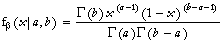where 0<=x<=1, a>=1, and b>=a+1. The gamma function,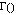, is a generalization of the factorial function. Factorials are only defined for integers, but the gamma function can have any number for an argument. If n is an integer,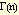= (n-1)!.

The equations for the mean and variance are: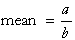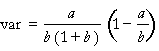The equations for the parameters are: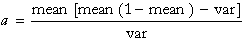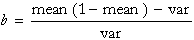The mean must be between 0 and 1. Also the expressions for a and b must produce positive numbers, which means the variance must be less than mean*(1-mean).

Versions: DPL Professional, DPL Enterprise, DPL Portfolio